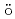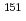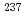# Benchmark study of DFT with Eu and Np Mssbauer isomer shifts using second-order Douglas-Kroll-Hess Hamiltonian
We optimized a mixing ratio of exchange energy between pure DFT and exact Hartree-Fock using TPSS exchange-correlation functional to estimate the accurate coordination bonds in f-block complexes by numerically benchmarking with the experimental data of Mssbauer isomer shifts forEu andNp nuclides. Second-order Douglas-Kroll-Hess Hamiltonian with segmented all-electron relativistically contracted basis set was employed to calculate the electron densities at Eu and Np nuclei, i.e. contact densities, for each five complexes for Eu(III) and Np(IV) systems. We compared the root mean square deviation values of their isomer shifts between experiment and calculation by changing the mixing ratio of Hartree-Fock exchange parameter from 0 to 100 % at intervals of 10 %. As the result, it was indicated that the mixing ratio of 30 and 60 % for Eu and Np benchmark systems, respectively, gives the smallest deviation values. Mulliken's spin population analysis indicated that the covalency in the metal-ligand bonds for both Eu and Np complexes decreases with increasing the Hartree-Fock exchange admixture.